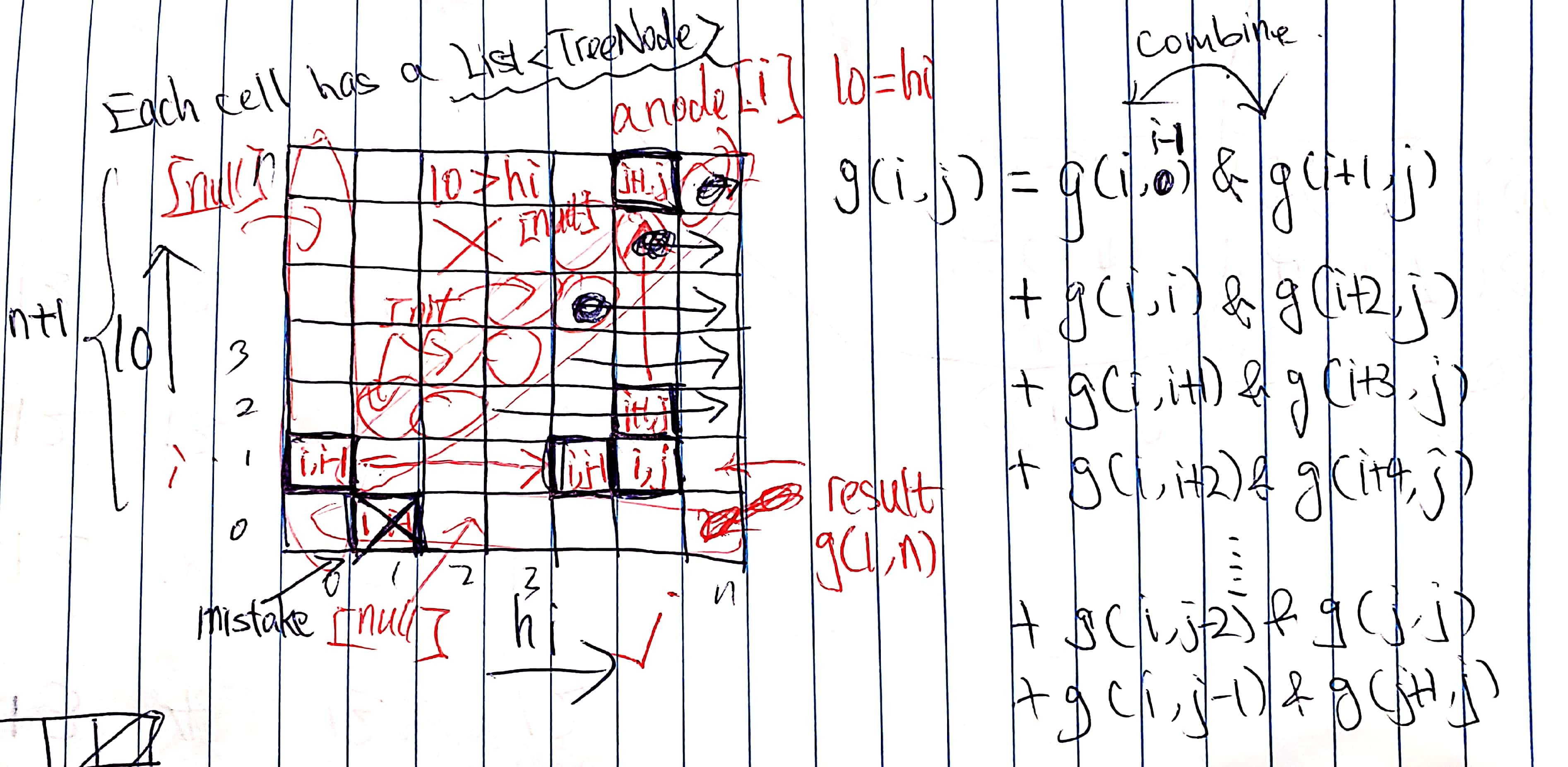# 95. Unique Binary Search Trees II

Reference: LeetCode
Difficulty: Medium

## Problem

Given an integer $n$, generate all structurally unique BST’s (binary search trees) that store values 1 ... n.

Example:

## Analysis

### Recursion

If we directly apply the solution in 96. Unique Binary Search Trees, we have an incorrect result. Consider the following example.

As you can see, although there are still 2 nodes in the right subtrees, the values are not the same. In the previous approach, it did not handle this situation.

So rather than passing a parameter n (number of nodes), we now pass down two parameters lo and hi indicating the range of values of a tree.

For example, generateTrees(1, 5) generate trees whose values range from 1 to 5, and each of them has a chance to be the root, which also includes the information of number of nodes n. Say when the root is 3, we calculate generateTrees(1, 2) and generateTrees(4, 5).

Note: When n == 0, it returns [] instead of [[null]].

Time: It is at most bounded by $O(N \times N!)$. A tighter bound would be Catalan number times $N$ since we’ve done $N$ times, which is $N \times G_N = O(N\times\frac{4^N}{N^{3/2}}) = O(\frac{4^N}{N^{1/2}})$.
Space: $O(N \times G_N) = O(\frac{4^N}{N^{1/2}})$

### DP (Clone)

Let’s denote tree[i] as the list of trees of size i. Think of tree[i] as our building blocks for a larger tree.

So we can compute all possible trees for tree, tree, …, then we can construct tree[n] by previous results.

For a small n = 3 , we notice that when we calculate tree we want all possible combinations for tree ([1 2], [2 3]). Furthermore, if we have a large n = 100, we want all the combinations as follows [1 2], [2 3], [3 4], …, [99 100] (each of them has two structures).

Since these trees have the same two types of structures:

We can actually construct all the trees by [1 2] plus some constant, say offset. For example, [5 6] can be constructed as follows:

Say the problem is n = 100. During the execution of the algorithm when i = 98, we want to get all possible trees for i = 98 as the root. The size of the left subtree is 97 and the subtree is picked from tree; the size of the right subtree is 2 and the subtree is picked from tree.

For the left subtree, we already have tree computed as [1 2 3 ... 97].

For the right subtree, we want [99 100], which can be computed by [1 2] plus offset = 98.

Therefore, given a tree root, we can generate a new tree by cloning with an offset.

For input n, the result we want is tree[n] ([1 2 3 ... n]). Here is the code for generateTrees(n):

Time: N/A (I don’t know T_T)
Space: N/A (I don’t know T_T)

### DP (2D)

Judge: 1ms, faster than 99.95%. I have nothing to say.Here is the code I wrote:

Time: N/A (I don’t know T_T)
Space: N/A (I don’t know T_T)

CommentJunhao Wang
a software engineering cat